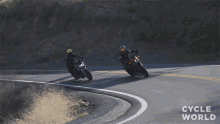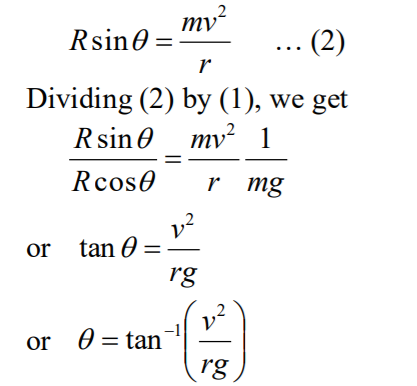⁕ Motion of a vehicle in a flat circular path↪ Suppose a car of mass m is moving in a flat circular path of radius r with a constant speed v.
↪ During the motion, the sideslip of the tyres is prevented by the forces of friction f1 and f2, which act toward the center of the circular path.
↪ If R1 and R2 are the normal reactions of the ground on the tyres, thenwhere μ is the coefficient of friction. The total force of friction provides the necessary centripetal force.
That is,As the total normal reaction = R1 + R2 = mg, thenThis gives a maximum velocity with which the car moves without side-slipping.

⁕ Banking of Tracks↪ If the outer edge of a circular track is raised above the inner edge by a certain height, then the track is called a banked track.
↪ The angle made by such a track with the horizontal is called a banking angle.

↪ Suppose a car of mass m is moving in a circular banked track of radius r with speed v.
↪ Let R be the normal reaction of the ground on the car and let θ be a banking angle and hence the angle between R and the vertical.
↪ The component of R along the vertical is balanced by the weight mg of the car.
That is,and the component of R along the horizontal provides the necessary centripetal force.
That is,↪ This gives a maximum banking angle of the track for no sideslip.
↪ So a racing-car track is shaped like a saucer just to prevent from side-slipping.↪ If the outer edge of the track is raised above the inner edge by a height h and d is the width of the track, thenIf θ is very small,
sinθ = tanθ = h/d.
Substituting the value of tanθ in (3), we get↪ Thus while constructing a banked track, the value of h is designed for the fixed values of v, d and r.

⁕ Bending of a cyclist↪ Suppose a cyclist of mass m (mass of the cyclist and the cycle) is moving in a curved path ofradius r with speed v.

↪ To provide the necessary centripetal force, the cyclist leans at an angle θ with the vertical.
↪ Let R be the normal reaction of the ground on the cyclist and hence θ is the angle between R and the vertical.
↪ The component of R along the vertical will balance the weight of the cyclist.
That is,

Rcosθ = mg … (1)

and the component of R along the horizontal provides the necessary centripetal force.
That is,↪ This gives the maximum angle at which the cyclist should lean with the vertical when he takes a turn in the curved path without falling.